# Proton charge Neutron neutral charge Electron charge Ions

• Slides: 15• Proton = + charge • Neutron = neutral charge • Electron = - chargeIons • Ions are atoms that have lost or gained one or more electrons giving them a positive or negative charge!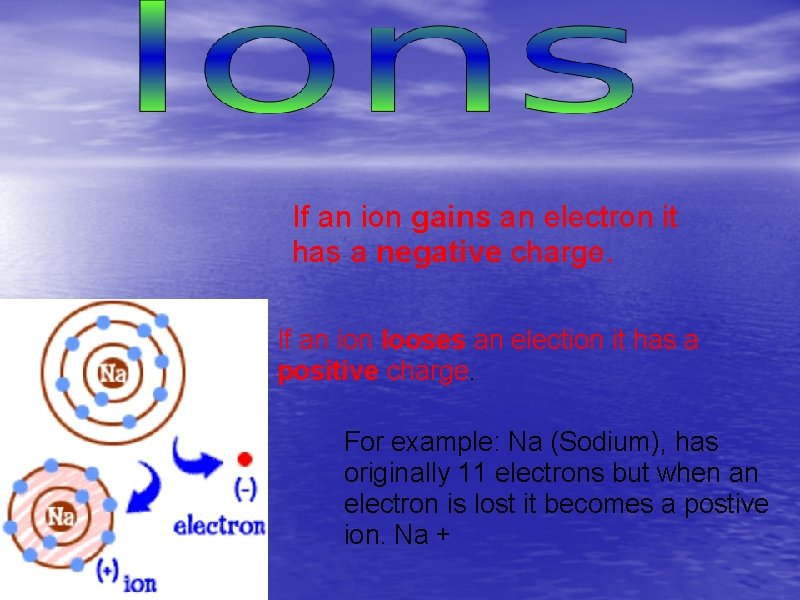If an ion gains an electron it has a negative charge. If an ion looses an election it has a positive charge. For example: Na (Sodium), has originally 11 electrons but when an electron is lost it becomes a postive ion. Na +What causes an atom to become an ion? • When an atom loses or gains one or more electrons it acquires a net electrical charge called an ion. • The net charge of an ion is found by subtracting the number of electrons from the number of protons Charge of Ion = number of protons – number of electrons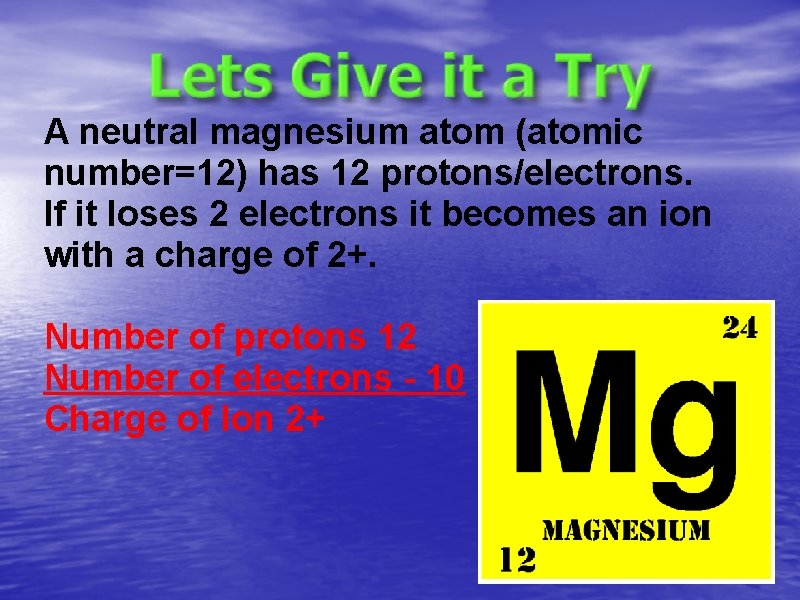A neutral magnesium atom (atomic number=12) has 12 protons/electrons. If it loses 2 electrons it becomes an ion with a charge of 2+. Number of protons 12 Number of electrons - 10 Charge of Ion 2+Ion 19. Cu²+ 20. F- Protons Electrons 29 27 9 10Isotopes • Isotopes are atoms that have the same number of protons but different numbers of neutrons • Most elements in the first two rows of the periodic table have at least 2 isotopes with one being more common than the other • In nature, elements are almost always found as a mixture of isotopes Hmm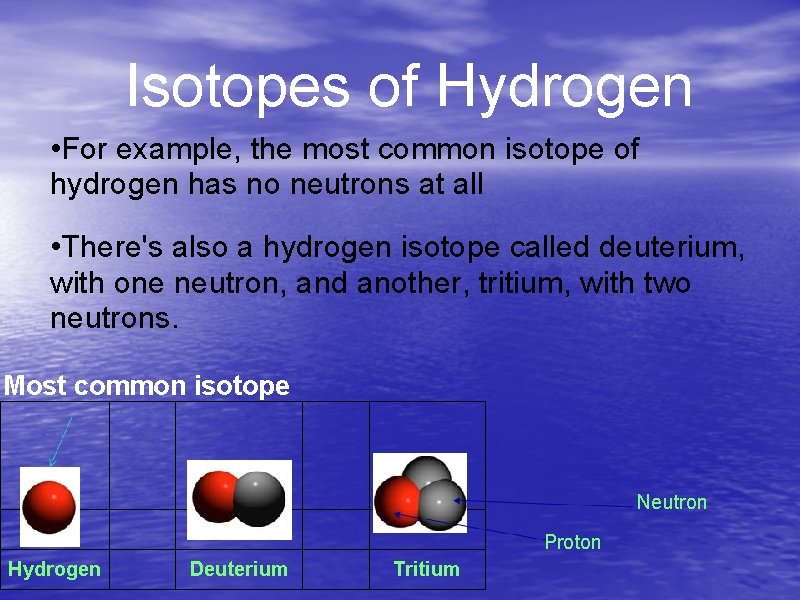Isotopes of Hydrogen • For example, the most common isotope of hydrogen has no neutrons at all • There's also a hydrogen isotope called deuterium, with one neutron, and another, tritium, with two neutrons. Most common isotope Neutron Proton Hydrogen Deuterium Tritium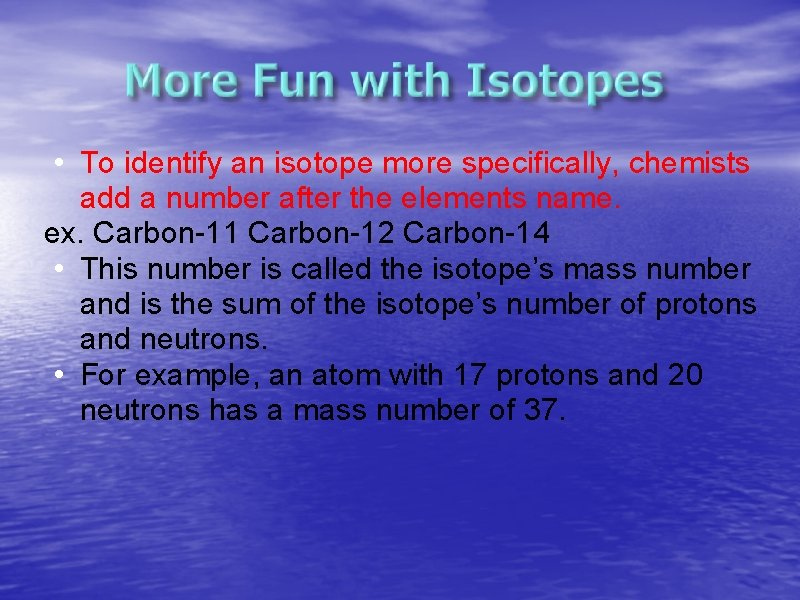• To identify an isotope more specifically, chemists add a number after the elements name. ex. Carbon-11 Carbon-12 Carbon-14 • This number is called the isotope’s mass number and is the sum of the isotope’s number of protons and neutrons. • For example, an atom with 17 protons and 20 neutrons has a mass number of 37.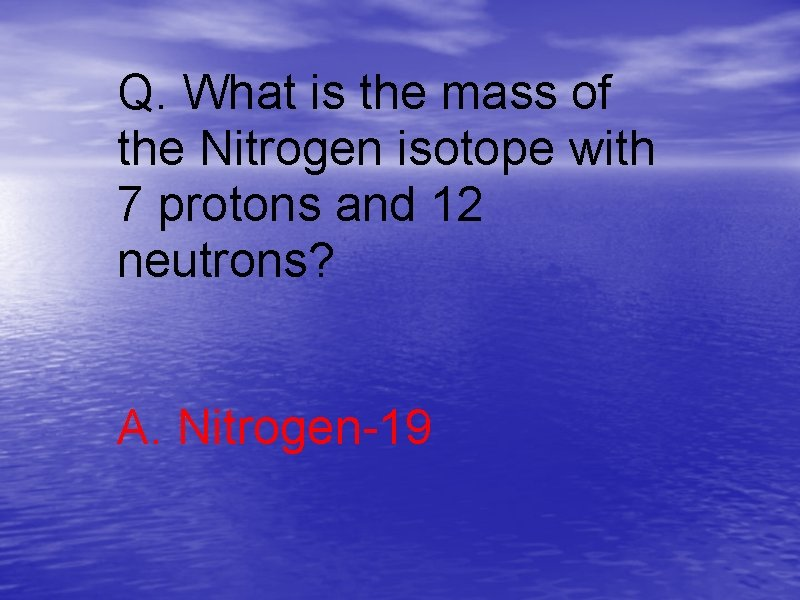Q. What is the mass of the Nitrogen isotope with 7 protons and 12 neutrons? A. Nitrogen-19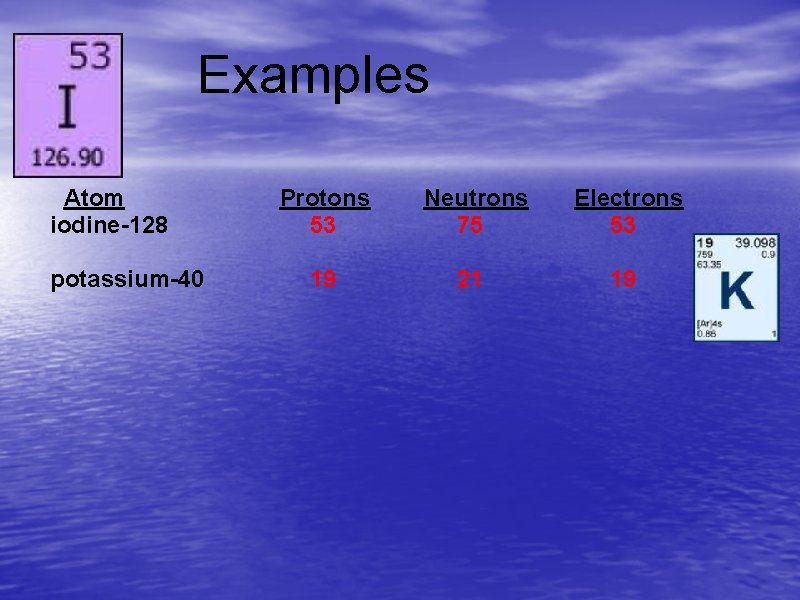Examples Atom iodine-128 potassium-40 Protons 53 Neutrons 75 Electrons 53 19 21 19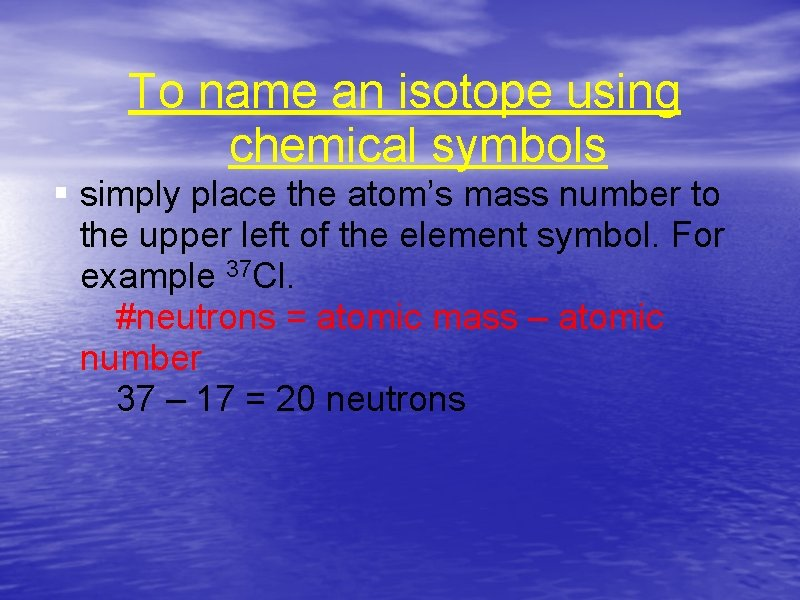To name an isotope using chemical symbols § simply place the atom’s mass number to the upper left of the element symbol. For example 37 Cl. #neutrons = atomic mass – atomic number 37 – 17 = 20 neutronsHow many protons, neutrons, and electrons are present? 56 26 Fe 2+ STEP 1: The atomic number in the lower left corner = 26 protons. The difference between the mass number and the atomic number is 56 – 26 = 30. There are 30 neutrons. STEP 2: The charge on the ion, 2+ shows us that there are 2 more protons than electrons, indicating that there are 24 electrons.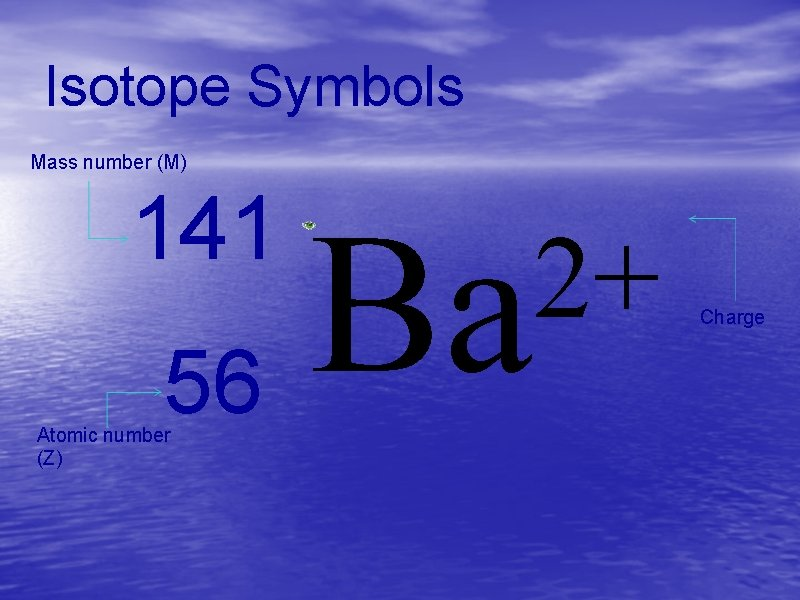Isotope Symbols Mass number (M) 141 Ba 56 Atomic number (Z) 2+ Charge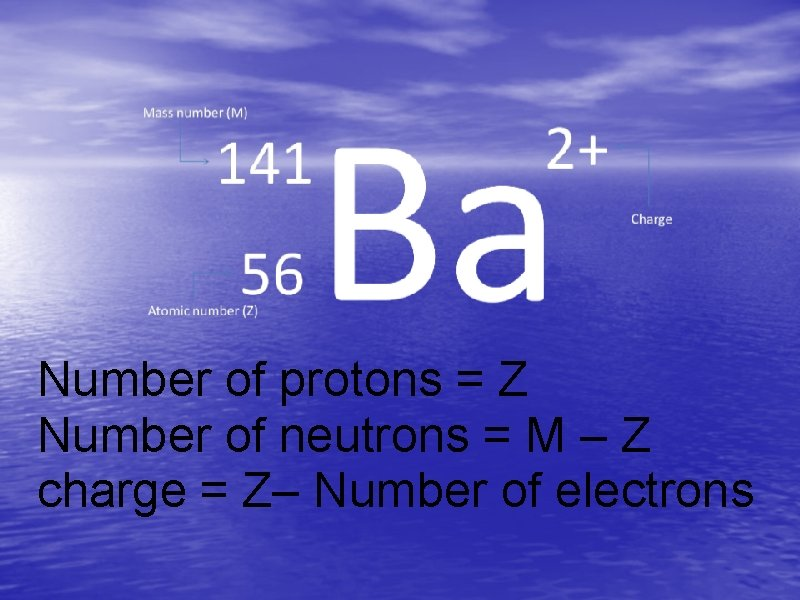Number of protons = Z Number of neutrons = M – Z charge = Z– Number of electrons